# Classification of terms for the balance sheet

• Study Notes
• Discuss This Topic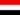FAISAL A. 0 0 It's there any examples for the classification of terms for the balance sheetFAISAL A. 0 0 What are the classification of terms for the balance sheet.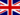Janet S. 1 0 I think it helps assess liquidity.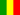Gabriel O. 1 0 The amount of current liabilities is used in two of the most common financial ratios. Working capital is the amount of current assets minus the amount of current liabilities. The current ratio is computed by dividing the amount of current assets by the amount of current liabilities.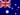Wendy C. 1 0 Classification of terms is necessary to understanding the balance sheet.Wendy C. 0 0 .Wendy C. 1 0 Classification of terms for the balance sheet Classification of items into current and non-current sections helps assess liquidity. There are three criteria for separating current from non-current assets: • Time - How long before the asset is expected to be converted into cash? • Intention - Is it intended to convert the asset into cash in the near future? • Economic benefit - For how long will the asset continue to earn revenue for the business? Classification of terms is necessary to understanding the balance sheet.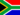Samuel F. 0 0 Why do you separate current liabilities from long-term liabilities?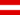Harrison A. 1 0 How do classification of items into current and non current sections helps assess liquidity ?Zachary B. 0 0 What is classification of terms for the balance sheet?
• Text Version